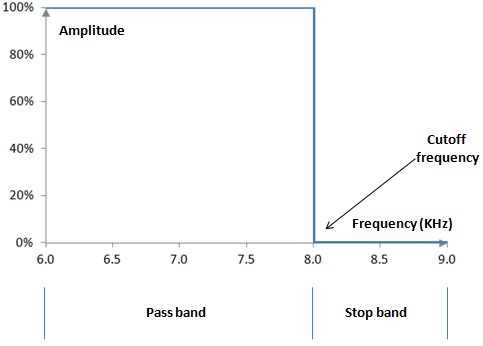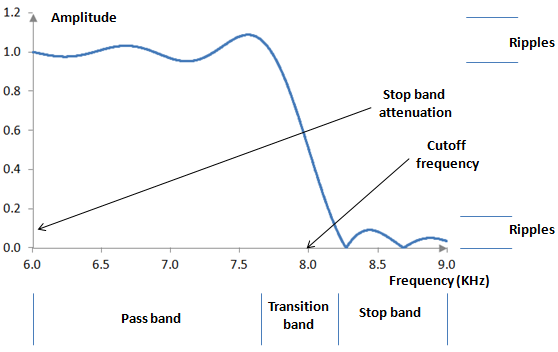# Magnitude response

In signal processing, the magnitude response of an analog or digital equipment or software is some representation, usually numeric, graphical, or mathematical, of how that equipment or software changes the amplitude of a set of frequencies. In most cases, the magnitude response is the ratio of the amplitude of frequencies in the output signal to the amplitude of frequencies of the input signal.

Usually, if we want to describe how a system impacts the amplitudes of frequencies in a signal, we will use the term magnitude response. If we want to describe how the system changes the phase of frequencies in the signal, we will use the term phase response. If we want to describe changes to both the amplitudes and phase of frequencies in the signal, we will use the term frequency response.

## Example: Magnitude response of an ideal low pass filter at 8 KHz

One would expect an ideal low pass filter to leave low frequencies unchanged (to "pass" them) and to significantly decrease the amplitude of high frequencies (to "stop" them). An ideal low pass filter then could have the magnitude response shown at the graph below.The "amplitude" in the graph above is the ratio of the output signal amplitude at a frequency to the input signal amplitude at the same frequency. 100% means that the filter leaves the amplitude of the specific frequency unchanged. 0% means that the specific frequency is effectively zeroed out or removed. This filter then leaves the amplitudes of the frequencies below some "cutoff" frequency (the "transition frequency", 8 KHz in this filter) unchanged, while it removes, or filters out, or stops, the frequencies that are higher than that frequency. The set of frequencies that are left unchanged are called the "pass band" of the filter. The set of frequencies that are removed are called the "stop band" of the filter.

Mathematically, the magnitude response H of this ideal low pass filter is a function of the incoming frequency f that looks something like the following.

$$|H(f)|=\begin{cases} 100\%, f \le transition frequency \\ 0\%, f \gt transition frequency \end{cases}$$

Other filters can have different magnitude responses. A high pass filter, for example, is expected to pass higher frequencies while stopping lower ones. A band pass filter is expected to pass only the frequencies in a certain frequency interval while stopping everything above or below the interval. A notch filter is expected to pass all frequencies but those in a very narrow frequency interval, and so on. An all pass filter is expected to leave the amplitudes of all frequencies unchanged (but change their phase, thus producing a specific phase response).

## Example: Magnitude response of a real digital low pass filter

The following picture shows the typical magnitude response of a digital low pass filter.The amplitude in this chart is simply the ratio of the output amplitude to the input amplitude for a selected frequency. It is not show as a percentage, as percentages are not the typical representation that is used. Most often, you can assume in a chart like that that the input amplitude of frequencies is 1 (i.e., it is normalized) and thus the output amplitude is whatever is shown on the graph.

This is not an ideal low pass filter and this magnitude response differs from the magnitude response of the ideal low pass filter. There is still a pass band – an interval of frequencies passed by the filter – and a stop band – an interval of frequencies stopped by the filter. There is, however, usually a transition band, as the filter does not immediately move from the pass band to the stop band, but rather does that smoothly over an interval of frequencies around the transition frequency. All pass band frequencies are not the same, as there are changes in the amplitude of those frequencies, which show up as "ripples" in the pass band. Digital filters characteristically have ripples that increase the closer we get to the transition band. Similarly, there are ripples in the stop band, which also increase the close we are to the transition band. The stop band frequencies are not completely "stopped" and so there is "stop band attenuation": the amount of decrease in the amplitude of the stop band frequencies.

The ripples in the pass band and in the stop band are characteristic of many, but not all filters. The Butterworth filter, for example, does not have ripples. The magnitude response of a Butterworth low pass filter is monotonically decreasing. All finite impulse response filters, on the other hand, have ripples, which are due to the Gibbs phenomenon – a manifestation of the fact that the Fourier series used to create these filters is a series of continuous functions, whereas the ideal magnitude response above is a discontinuous (although continuously differentiable) function.

The same magnitude response above can be shown in decibels as decibels simply measure the ratio of two quantities. In this case the decibel will measure the ratio of the output and input amplitudes. The following is the magnitude response of the same filter, but in decibels.## Example: Magnitude response and transfer functions

Given a discrete-time transfer function H(z) , computed with the Z transform, of some linear time-invariant system, the magnitude response of the system is given by

$$|H(e^{j \omega})|=\sqrt{(Re(H(e^{j \omega})))^2+(Im(H(e^{j \omega})))^2}$$

where Re(H) and Im(H) are the real and imaginary parts of the complex number H. (The continuous-time equivalent would be |H(jω)|; see Frequency response for more detail). Say that this is a finite impulse response digital filter with length N and weights a(k) and a transfer function H(z). The magnitude response of this filter can be computed with the following formula.

$$|H(f)|=\sqrt{(\sum_{k=0}^{N-1} a(k) \, \cos(\frac{2\pi k f}{f_s}))^2+(\sum_{k=0}^{N-1} a(k) \, \sin(\frac{2\pi k f}{f_s}))^2}$$

Here H(f) is the magnitude response at frequency f, a(k) are the weights of the filter at samples k = 0,…, N-1, and fs is the sampling frequency. (Setting z = e in the Z transform produces the discrete-time Fourier transform. Euler's formula gives us e = cos(ω) + j sin(ω). The angular frequency ω can be replaced with ω = 2 π f / fs).

### Filtered HTML

• Freelinking helps you easily create HTML links. Links take the form of [[indicator:target|Title]]. By default (no indicator): Click to view a local node.